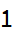# BitFlip

Flip an individual bit in an integer (0 to 1, and 1 to 0)

 ResourceFunction["BitFlip"][n,p] flips the pth bit of integer n.

## Details and Options

The lowest bit of integer n (e.g. signifying 20) is flipped with p=0.

## Examples

### Basic Examples

Flip the 0th bit of the number 2, from a zero to a one:

 In:=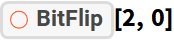Out=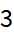Flip the 0th bit of the number 3, from a one to a zero:

 In:=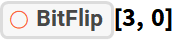Out=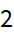Starting with the number zero, flip positional bits:

 In:=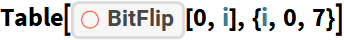Out=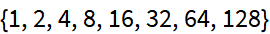### Scope

Flip the bit associated with 24:

 In:=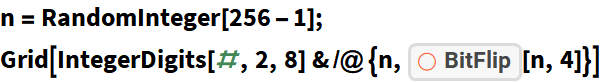Out=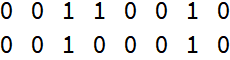Flip the bit associated with 27:

 In:=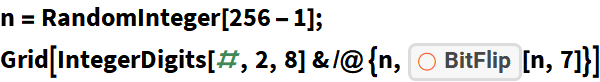Out=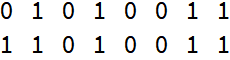### Applications

Compute the Hamming-distance between a random integer and the same number with a single random bit flipped:

 In:=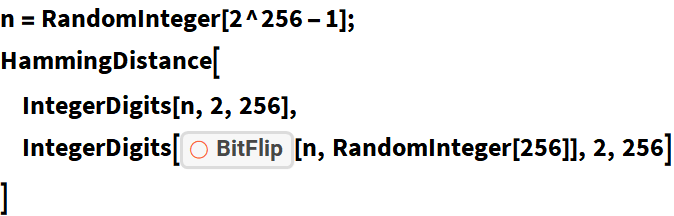Out=